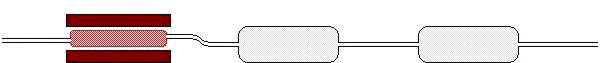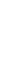Java Security Settings:
This web page employs Java, which requires specific security settings for correct operation.
If the applets on this page do not run correctly, consult the Virtual Chemistry Experiments FAQ
or the Physlet Physics web site for establishing the correct security settings.

Stoichiometry

Elemental Analysis: Carbon and Hydrogen

Composition by Mass

The composition of a compound is often expressed in terms of the weight percent of each element in the compound.

For example, ethanol has the formula C2H6O. One mole of ethanol has a mass of 46.07 g. The elemental formula indicates that one mole of ethanol contains two moles of carbon, six moles of hydrogen, and one mole of oxygen. Thus the composition of the compound by mass is

 % C = 2 moles C (12.01 g/mole C)46.07 g ethanol 100 % = 52.14 %

Similarly the weight percents of hydrogen and oxygen in ethanol are

 % H = 6 moles H (1.008 g/mole H)46.07 g ethanol 100 % = 13.13 %

 % O = 1 mole O (16.00 g/mole O)46.07 g ethanol 100 % = 34.73 %

Notice that the sum of the weight percents of all the elements in a compound must equal 100 %.

52.14 % + 13.13 % + 34.73 % = 100.00 %

Elemental Analysis

Elemental analysis is an experiment that determines the amount (typically a weight percent) of an element in a compound. Just as there are many different elements, there are many different experimental methods for determining elemental composition. The most common type of elemental analysis is for carbon, hydrogen, and nitrogen (CHN analysis). This type of analysis is especially useful for organic compounds (compounds containing carbon-carbon bonds).

The elemental analysis of a compound enables one to determine the empirical formula of the compound. The empirical formula is the formula for a compound that contains the smallest set of integer ratios for the elements in the compound that gives the correct elemental composition by mass.

Consider the compound octane, whose molecular formula is C8H18. The ratio of carbon to hydrogen atoms in octane is 8 : 18. This ratio has a value of 0.444444, which can also be expressed by ratios such as 16 : 36 and 1 : 2.25. The smallest ratio of integers that gives this ratio is 4 : 9 and therefore the empirical formula of octane is C4H9.

The molecular formula is an integer (n) multiple of the empirical formula. For octane the empirical formula is C4H9; thus the molecular formula is C4nH9n. In this case n = 2, which produces C8H18.

If one prepares or isolates a new compound, the determination of the molecular formula for the compound is an important goal. This determination typically involves two steps: (1) elemental analysis of the compound and (2) determination of the molecular mass. The elemental analysis permits determination of the empirical formula, and the molecular weight and elemental analysis permit determination of the molecular formula.

To illustrate this process, consider an unknown compound containing only carbon and hydrogen whose elemental composition is 84.72% carbon and 15.41% hydrogen by weight. (Notice that the experimental error has produced values whose sum is not exactly 100.00 %.) Suppose one had a 100.0 g sample of this compound. (Any amount could be chosen; 100.0 g just happens to be a convenient number to use.) The 100.0 g sample of the compound contains 100.0 g (0.8472) = 84.72 g carbon and 100.0 g (0.1541) = 15.41 g hydrogen.

These amounts can be expressed in terms of moles of each element.

 84.72 g C 1 mole C12.01 g C = 7.054 mole C

 15.41 g H 1 mole H1.008 g H = 15.29 mole H

The atom ratio C : H is thus 7.054 : 15.29 or C7.054H15.29. Of course formulas are generally expressed in terms of integers, and the empirical formula is the smallest ratio of integers that yields the correct atom ratio. There are several ways to arrive at the correct set of integers. If the molecule is reasonably small, trial and error is frequently the most direct approach.

First divide each term in the ratio by the smallest value (7.054 in this case).

15.29 / 7.054 = 2.168, thus the ratio 7.054 : 15.29 is the same as 1.000 : 2.168

In this case, the integer for C cannot be 1, because this integer does not lead to an integer value for H. Keep multiplying this ratio by small integers (2, 3, 4, ...) until a ratio of integers is obtained.

2.000 : 4.336

3.000 : 6.504

4.000 : 8.672

5.000 : 10.84

6.000 : 13.01

In this case, the smallest ratio of integers that produces 2.182 for C : H is 6 : 13 and thus the empirical formula is C6H13.

Be aware that the elemental analysis is not perfectly accurate. The experimental error will generally produces atom ratios that are not perfect integers but are close to integers.

A separate measurement of the molecular mass is required to determine the molecular formula. For this compound mass spectrometry gave a molecular mass of 170.

The molecular formula is C6nH13n, thus the molecular mass is

170. = 12.01 ( 6 n ) + 1.008 ( 13 n ) = 85.164 n

n = 170./85.164 = 1.996 or 2 (bear in mind n must be an integer)

The molecular formula is thus C12H26.

Experiment

Objectives

• Determine the % C and % H in an unknown compound.
• Determine the empirical formula of the unknown compound.
• Determine the molecular formula of the unknown compound.

Carbon-Hydrogen (CH) analysis is performed by burning the unknown sample. As a result of the complete combustion of the compound, all of the carbon in the compound is converted to carbon dioxide gas and all of the hydrogen in the compound is converted to water vapor.

CxHyA + z O2 (g)   →   x CO2 (g) + y/2 H2O (g) + A

The combustion is conducted in the presence of excess oxygen ( z >> x + y/2 ). The symbol A represents other elements in the system, which burn to produce either a solid residue or an unreactive gas (unreactive for the purposes of this analysis).

The sample is typically wrapped in a tin capsule and inserted into a furnace held at 1200oC. A steady stream of an unreactive gas (e.g., helium) is passed through the furnace and a burst of pure oxygen gas is added as the sample capsule is inserted into the oven. As the sample burns, the temperature rises to approximately 1700oC. The furnace contains a catalyst (Cr2O3) that facilitates the complete combustion of the sample. Copper turnings are also present to ensure that any nitrogen in the sample is reduced to N2, which is unreactive.

As the gas leaves the furnace, the carbon dioxide and water vapor produced by the combustion reaction are carried out of the furnace. The gas stream first passes through a desiccant, typically Mg(ClO4)2, which removes all moisture from the gas stream. Next the gas stream passes through a tube containing NaOH, which removes all carbon dioxide from the gas stream (forming NaHCO3).

By weighing the tubes containing Mg(ClO4)2 and NaOH before and after the combustion reaction, it is possible to determine the amounts of water and carbon dioxide produced by the combustion reaction and thus the amounts of hydrogen and carbon in the unknown compound.

The experimental apparatus is shown in the image below. The furnace lies at the left side of the image. The tubes containing Mg(ClO4)2 (center of the image) and NaOH (right side of the image) rest on balances, which permit their masses to be determined. (The gas line connecting the two tubes is very light and flexible. For the purposes of this simulation, it is assumed they do not perturb the measurements of the masses of the tubes.)

The experimental procedure is as follows.

1. Select an unknown compound (A, B, C, D, or Random).

Unknowns A, B, C, and D are specific compounds; you will not be given the actual empirical and molecular formulas for these compounds. The Random unknown varies from one experiment to the next, and you may see the empirical and molecular formulas for this compound. The Random unknown is useful for practicing calculations for this type of analysis. (Even though the Random option is selected, clicking on the option again will select a new compound.)
Each compound contains carbon and hydrogen and possibly one other element. Think about how you might determine the weight percent for this third element.

2. Zero the readings on the balances.

To make calculations easier, click the "Tare" button on each balance to zero the mass reading. Any increase in mass during the experiment will thus be attributable to the adsorption of water or carbon dioxide from the combustion of the sample.

3. Initiate the combustion reaction by selecting the "Burn Sample" button.

4. Wait a few seconds for the combustion reaction to occur, the water and carbon dioxide to be absorbed, and the mass readings to stabilize.

5. Record the masses of water and carbon dioxide produced by the combustion of the sample.

6. Calculate the empirical formula for the unknown compound. The molecular mass of the compound is provided; use this value and the empirical formula to determine the molecular formula for the unknown compound. (In this experiment, the molecular mass is obtained from low-resolution mass spectrometry and is thus known to the nearest amu.)

 Furnace H2O Trap CO2 TrapSelect Sample A B C D Random Compound Contains Molecular Mass Mass of Sample gStoichiometry Home PageVirtual Chemistry Home Page

CH.html version 2.11